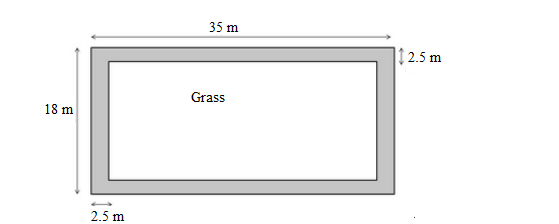# A rectangular park 35 m long 18 m wide is to be covered with grass, leaving 2.5 m uncovered all around it.`
Question:

A rectangular park 35 m long 18 m wide is to be covered with grass, leaving 2.5 m uncovered all around it. Find the area to be laid with grass.

Solution:

The field is planted with grass, with 2.5 m uncovered on its sides.

The field is shown in the given figure.Thus, we have;

Length of the area planted with grass $=35-(2.5+2.5)=35-5=30 \mathrm{~m}$

Width of the area planted with grass $=18-(2.5+2.5)=18-5=13 \mathrm{~m}$

Area of the rectangular region planted with grass $=30 \times 13=390 \mathrm{~m}^{2}$